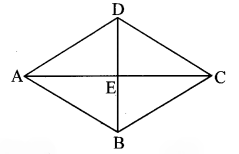# Maharashtra Board 8th Class Maths Practice Set 15.2 Solutions Chapter 15 Area

Balbharti Maharashtra State Board Class 8 Maths Solutions covers the Practice Set 15.2 8th Std Maths Answers Solutions Chapter 15 Area.

## Practice Set 15.2 8th Std Maths Answers Chapter 15 Area

Question 1.
Lengths of the diagonals of a rhombus are 15 cm and 24 cm, find its area.
Solution:
Lengths of the diagonals of a rhombus are 15 cm and 24 cm.
Area of a rhombus
= $$\frac { 1 }{ 2 }$$ × product of lengths of diagonals
= $$\frac { 1 }{ 2 }$$ × 15 × 24
= 15 × 12
= 180 sq.cm
∴ The area of the rhombus is 180 sq. cm.

Question 2.
Lengths of the diagonals of a rhombus are 16.5 cm and 14.2 cm, find its area.
Solution:
Lengths of the diagonals of a rhombus are 16.5 cm and 14.2 cm.
Area of a rhombus
= $$\frac { 1 }{ 2 }$$ × product of lengths of diagonals
= $$\frac { 1 }{ 2 }$$ × 16.5 × 14.2
= 16.5 × 7.1
= 117.15 sq cm
∴ The area of the rhombus is 117.15 sq. cm.

Question 3.
If perimeter of a rhombus is 100 cm and length of one diagonal is 48 cm, what is the area of the quadrilateral?
Solution:
Let ₹ABCD be the rhombus. Diagonals AC and BD intersect at point E.l(AC) = 48 cm …(i)
l(AE) = $$\frac { 1 }{ 2 }l(AC)$$ …[Diagonals of a rhombus bisect each other]
= $$\frac { 1 }{ 2 }$$ × 48 …[From (i)]
= 24 cm …(ii)
Perimeter of rhombus = 100 cm …[Given]
Perimeter of rhombus = 4 × side
∴ 100 = 4 × l(AD)
∴ l(AD) = $$\frac { 100 }{ 4 }$$ = 25 cm …(iii)
m∠AED = 90° …[Diagonals of a rhombus are perpendicular to each other]
∴ [l(AD)]² = [l(AE)]² + [l(DE)]² … [Pythagoras theorem]
∴ (25)² = (24)² + l(DE)² … [From (ii) and (iii)]
∴ 625 = 576 + l(DE)²
∴ l(DE)² = 625 – 576
∴ l(DE)² = 49
∴ l(DE) = √49
… [Taking square root of both sides]
l(DE) = 7 cm …(iv)
l(DE) = $$\frac { 1 }{ 2 } l(BD)$$ ….[Diagonals of a rhombus bisect each other]
∴ 7 = $$\frac { 1 }{ 2 } l(BD)$$ …[From (iv)]
∴ l(BD) = 7 × 2
= 14 cm …(v)
Area of a rhombus
= $$\frac { 1 }{ 2 }$$ × product of lengths of diagonals
= $$\frac { 1 }{ 2 }$$ × l(AC) × l(BD)
= $$\frac { 1 }{ 2 }$$ × 48 × 14 … [From (i) and (v)]
= 48 × 7
= 336 sq.cm
∴ The area of the quadrilateral is 336 sq.cm.

Question 4.
If length of a diagonal of a rhombus is 30 cm and its area is 240 sq.cm, find its perimeter.
Solution:
Let ₹ABCD be the rhombus.
Diagonals AC and BD intersect at point E.
l(AC) = 30 cm …(i)
and A(₹ABCD) = 240 sq. cm .. .(ii)Area of the rhombus = $$\frac { 1 }{ 2 }$$ × product of lengths of diagonal
∴ 240 = $$\frac { 1 }{ 2 }$$ × l(AC) x l(BD) …[From (ii)]
∴ 240 = $$\frac { 1 }{ 2 }$$ × 30 × l(BD) …[From (i)]
∴ l(BD) = $$\frac { 240\times 2 }{ 30 }$$
∴ l(BD) = 8 × 2 = 16 cm …(iii)
Diagonals of a rhombus bisect each other.
∴ l(AE) = $$\frac { 1 }{ 2 }l(AC)$$
= $$\frac { 1 }{ 2 }$$ × 30 … [From (i)]
= 15 cm …(iv)
and l(DE) = $$\frac { 1 }{ 2 }l(BD)$$
= $$\frac { 1 }{ 2 }$$ × 16
= 8 cm
m∠AED = 90°
…[Diagonals of a rhombus are perpendicular to each other]
…[Pythagoras theorem]
∴l(AD)² = (15)² + (8)² … [From (iv) and (v)]
= 225 + 64